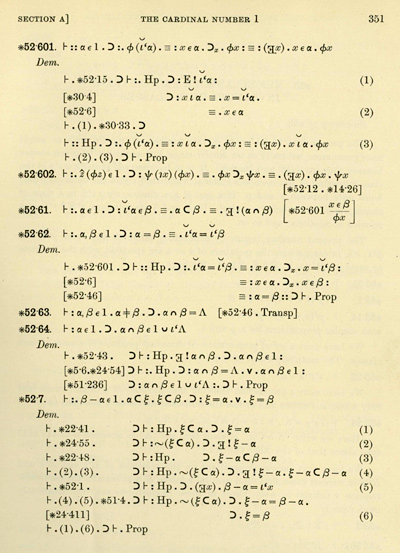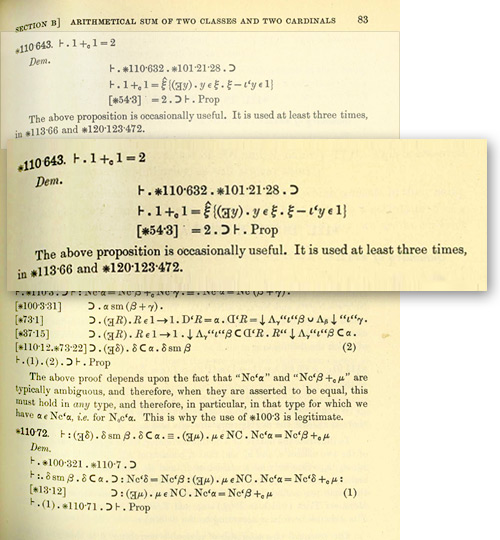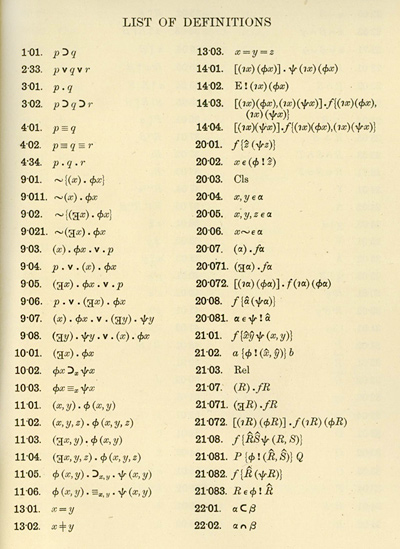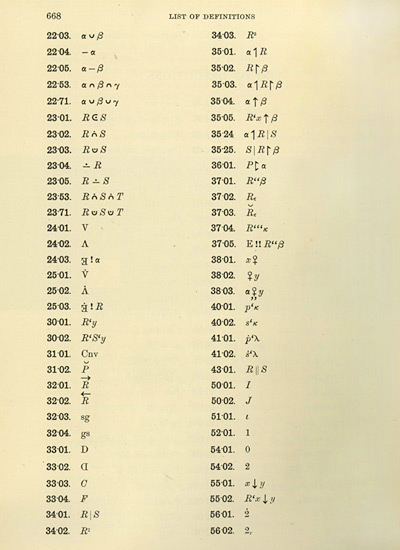# 100 Years Since Principia Mathematica

A hundred years ago this month the first volume of Whitehead and Russell’s nearly-2000-page monumental work Principia Mathematica was published. A decade in the making, it contained page after page like the one below, devoted to showing how the truths of mathematics could be derived from logic.Principia Mathematica is inspiring for the obvious effort put into it—and as someone who has spent much of their life engaged in very large intellectual projects, I feel a certain sympathy towards it.

This essay is also in Idea Makers: Personal Perspectives on the Lives & Ideas of Some Notable People »

For additional perspectives on related topics see “Logic, Explainability and the Future of Understanding” (2018) »

In my own work, Mathematica shares with Principia Mathematica the goal of formalizing mathematics—but by building on the concept of computation, it takes a rather different approach, with a quite different outcome. And in A New Kind of Science, one of my objectives, also like Principia Mathematica, was to understand what lies beneath mathematics—though again my conclusion is quite different from Principia Mathematica.

Ever since Euclid there had been the notion of mathematical proof as a formal activity. But there had always been a tacit assumption that mathematics—with its numbers and geometrical figures—was still at some level talking about things in the natural world.

In the mid-1800s, however, that began to change, notably with the introduction of non-Euclidean geometries and algebras other than those of ordinary numbers. And by the end of the 1800s, there was a general movement towards thinking of mathematics as abstract formalism, independent of the natural world.

Meanwhile, ever since Aristotle, there had in a sense been another kind of formalism—logic—which was originally intended to represent specific kinds of idealized human arguments, but had gradually become assumed to represent any valid form of reasoning.  For most of its history, logic had been studied, and taught, quite separately from mathematics. But in the 1800s, there began to be connections.

George Boole showed how basic logic could be formulated in algebraic terms (“Boolean algebra”). And then Gottlob Frege, working in some isolation in Germany, developed predicate logic (“for all”, “there exists”, etc.), and used a version of set theory to try to describe numbers and mathematics in purely logical terms.

And it was into this context that Principia Mathematica was born. Its two authors brought different things to the project. Alfred North Whitehead was an established Cambridge academic, who, in 1898, at the age of 38, had published A Treatise on Universal Algebra “to present a thorough investigation of the various systems of Symbolic Reasoning allied to ordinary Algebra”. The book discussed Boolean algebra, quaternions and the theory of matrices—using them as the basis for a clean, if fairly traditional, treatment of topics in algebra and geometry.

Bertrand Russell was a decade younger. He had studied mathematics as an undergraduate in Cambridge, and by 1900,  at the age of 28, he had already published books ranging in topic from German social democracy, to foundations of geometry, and the philosophy of Leibniz.

The nature of mathematics and mathematical truth was a common subject of debate among philosophers—-as it had been at some level since Plato—and Russell seems to have believed that by making use of the latest advances, he could once and for all resolve the debates. In 1903, he published The Principles of Mathematics, volume 1 (no volume 2 was ever published)—in essence a survey, without formalism, of how standard areas of mathematics could be viewed in logical terms.

His basic concept was that by tightening up all relevant definitions using logic, it should be possible to derive every part of mathematics in a rigorous way, and thereby immediately answer questions about its nature and philosophy. But in 1901, as he tried to understand the concept of infinity in logical terms, and thought about ancient logical problems like the liar’s paradox (“this statement is false”), he came across what seemed to be a fundamental inconsistency: a paradox of self reference (“Russell’s Paradox”) about whether the set of all sets that do not contain themselves in fact contains itself.

To resolve this, Russell introduced what is often viewed as his most original contribution to mathematical logic: his theory of types—which in essence tries to distinguish between sets, sets of sets, etc. by considering them to be of different “types”, and then restricts how they can be combined. I must say that I consider types to be something of a hack. And indeed I have always felt that the related idea of “data types” has very much served to hold up the long-term development of  programming languages. (Mathematica, for example, gets great flexibility precisely from avoiding the use of types—even if internally it does use something like them to achieve various practical efficiencies.)

But back around 1900, as both Russell and Whitehead were trying to extend their formalizations of mathematics, they decided to launch into the project that would consume a decade of their lives, and become Principia Mathematica.

Particularly since the work of Gottfried Leibniz in the late 1600s, there had been discussion of developing a notation for mathematics that transcended the imprecision of human language.  In 1879 Gottlob Frege introduced his Begriffsschrift (“concept script”)—which was a major advance in concepts and functionality, but had a strange two-dimensional layout that was almost impossible to read, or to print economically. And in the 1880s, Giuseppe Peano developed a cleaner and more linear notation, much of which is still in use today.

It did not help the dissemination of Peano’s work that he chose to write his narrative text in a language of his own construction (based on classical Latin) called Interlingua. But still, in 1900, Russell went to a back-to-back pair of conferences in Paris about philosophy and mathematics (notable for being where Hilbert announced his problems), met Peano and became convinced that his attempt to formalize mathematics should be based on Peano’s notation and approach. (Russell gave a distinctly pre-relativistic philosophical talk at the conference about absolute ordering of spatiotemporal events, while his wife Alys gave a talk about the education of women.)

The idea that mathematics could be built up from a small initial set of axioms had existed since the time of Euclid. But Russell and Whitehead wanted to have the smallest possible set, and have them be based not on ideas derived from observing the natural world, but instead on what they felt was the more solid and universal ground of logic.

With present-day experience of computers and programming it does not seem surprising that with enough “code” one should be able to start from basic concepts of logic and sets, and successfully build up numbers and the other standard constructs of mathematics. And indeed Frege, Peano and others had already started this process before 1900. But by its very weightiness, Principia Mathematica made the point seem both surprising and impactful.

Of course, it did not hurt the whole impression that it took until more than 80 pages into volume 2 to be able to prove (as “Proposition *110.643”) that 1+1=2 (with the comment “[This] proposition is occasionally useful”).I do not know if Russell and Whitehead intended Principia Mathematica to be readable by humans—but in the end Russell estimated years later that only perhaps 6 people had ever read the whole thing. To modern eyes, the use of Peano’s dot notation instead of parentheses is particularly difficult. And then there is the matter of definitions.

At the end of volume 1, Principia Mathematica lists about 500 “definitions”, each with a special notation. In many ways, these are the analogs of the built-in functions of Mathematica.  But in Principia Mathematica, instead of being given English-based names, all these objects are assigned special notations. The first handful are not too difficult to understand. But by the second page one’s seeing all sorts of strange glyphs, and I, at least, lose hope of ever being able to decipher what is written in them.Beyond these notational issues, there is a much more fundamental difference between the formalization of mathematics in Principia Mathematica and in Mathematica. For in Principia Mathematica the objective is to exhibit true theorems of mathematics, and to represent the processes involved in proving them. But in Mathematica, the objective is instead to compute: to take mathematical expressions, and evaluate them.

(These differences in objective lead to many differences in character. For example, Principia Mathematica is constantly trying to give constraints that indirectly specify whatever structure it wants to talk about. In Mathematica, the whole idea is to have explicit symbolic structures that can then be computed with.)

In the hundred years since Principia Mathematica, there has been slow progress in presenting theorems of mathematics in formal ways. But the idea of mathematical computation has taken off spectacularly—and has transformed the use of mathematics, and many areas of its development.

But what about the conceptual purposes of Principia Mathematica? Russell explained in the introduction to his The Principles of Mathematics that he intended to “reduce the whole of [the propositions of mathematics] to certain fundamental notions of logic”. Indeed, he even made what he considered to be a very general definition of “pure mathematics”  as all true logical statements that contain only variables like p and q, and not literals like “the city of New York”. (Applied mathematics, he suggested, would come from replacing the variables by literals.)

But why start from logic? I think Russell just assumed that logic was the most fundamental possible thing—the ultimate incontrovertible representation for all formal processes. Traditional mathematical constructs—like numbers and space—he imagined were associated with the particulars of our world. But logic, he imagined, was a kind of “pure thought”, and something more general, and wholly independent of the particulars of our world.

In my own work leading up to A New Kind of Science, I started by studying the natural world, yet found myself increasingly being led to generalize beyond traditional mathematical constructs. But I did not wind up with logic. Instead, I began to consider all possible kinds of rules—or as I have tended to describe it (making use of modern experience), the computational universe of all possible programs.

Some of these programs describe parts of the natural world. Some give us interesting fodder for technology. And some correspond to traditional formal systems like logic and mathematics.

One thing to do is to look at the space of all possible axiom systems. There are some technical issues about modern equational systems compared to implicational systems of the kind considered in Principia Mathematica. But the essential result is that dotted around the space of all possible axiom systems are the particular axiom systems that have historically arisen in the development of mathematics and related fields.

Is logic somehow special? I think not.

In Principia Mathematica, Russell and Whitehead originally defined logic using a fairly complicated traditional set of axioms. In the second edition of the book, they made a point of noting that by writing everything in terms of Nand (Sheffer stroke) rather than And, Or and Not, it is possible to use a much simpler axiom system.

In 2000, by doing a search of the space of possible axiom systems, I was able to find the very simplest (equational) axiom system for standard propositional logic: just the single axiom ((a.b).c).(a.((a.c).a)) = c. And from this result, we can tell where logic lies in the space of possible formal systems: in a natural enumeration of axiom systems in order of size, it is about the 50,000th formal system that one would encounter.

A few other traditional areas of mathematics—like group theory—occur in a comparable place. But most require much larger axiom systems.  And in the end the picture seems very different from the one Russell and Whitehead imagined. It is not that logic—as conceived by human thought—is at the root of everything.  Instead, there are a multitude of possible formal systems, some picked by the natural world, some picked by historical developments in mathematics, but most out there uninvestigated.

In writing Principia Mathematica, one of Russell’s principal objectives was to give evidence that all of mathematics really could be derived from logic. And indeed the very heft of the book gave immediate support to this idea, and gave such credibility to logic (and to Russell) that Russell was able to spend much of the rest of his long life confidently presenting logic as a successful way to address moral, social and political issues.

Of course, in 1931 Kurt Godel showed that no finite system—logic or anything else—can be used to derive all of mathematics. And indeed the very title of his paper refers to the incompleteness of none other than the formal system of Principia Mathematica. By this time, however, both Russell and Whitehead had moved on to other pursuits, and neither returned to address the implications of Godel’s Theorem for their project.

So can one say that the idea of logic somehow underlying mathematics is wrong? At a conceptual level, I think so. But in a strange twist of history, logic is currently precisely what is actually used to implement mathematics.

For inside all current computers are circuits consisting of millions of logic gates—each typically performing a Nand operation. And so, for example, when Mathematica runs on a computer, and implements the operations of mathematics, it does so precisely by marshalling the logic operations in the hardware of the computer. (To be clear, the logic implemented by computers is basic, propositional, logic—not the more elaborate predicate logic, combined with set theory, that Principia Mathematica ultimately uses.)

We know from computational universality—and more precisely from the Principle of Computational Equivalence—that things do not have to work this way, and that there are many very different bases for computation that could be used.  And indeed, as computers move to a molecular scale, standard logic will most likely no longer be the most convenient basis to use.

But so why is logic used in today’s computers? I suspect it actually has quite a bit to do with none other than Principia Mathematica. For historically Principia Mathematica did much to promote the importance and primacy of logic, and the glow that it left is in many ways still with us today. It is just that we now understand that logic is just one possible basis for what we can do—not the only conceivable one.

(People familiar with technical aspects of logic may protest that the notion of “truth” is somehow intimately tied to traditional logic.  I think this is a matter of definition, but in any case, what has become clear for mathematics is that it is vastly more important to compute answers than merely to state truths.)

A hundred years after Principia Mathematica there is still much that we do not understand even about basic questions in the foundations of mathematics. And it is humbling to wonder what progress could be made over the next hundred years.

When we look at Principia Mathematica, it emphasizes exhibiting particular truths of mathematics that its authors derived. But today Mathematica in effect every day automatically delivers millions of truths of mathematics, made to order for a multitude of particular purposes.

Yet it is still the case that it operates with just a few formal systems that happen to have been studied in mathematics or elsewhere. And even in A New Kind of Science, I concentrated on particular programs or systems that for one reason or another I thought were interesting.

In the future, however, I suspect that there will be another level of automation. Probably it will take much less than a hundred years, but in time it will become commonplace not just to make computations to order, but to make to order the very systems on which those computations are based—in effect in the blink of an eye inventing and developing something like a whole Principia Mathematica to respond to some particular purpose.